# An Intuitive Approach to Q-Learning (P1)

Note, the following articles will not be the usual step-by-step coding tutorials. Instead, we will focus more on acquiring a conceptual understanding by diving into the math of Q-learning in a three-part series. That being said, a basic understanding of probability and Markov chains will be useful in order to understand the following concepts. If you aren’t familiar with these concepts, watch this MIT series to get up to speed!

Usually, when I program a Machine Learning algorithm, I’m bothered by the fact that I don’t know the fundamental concepts behind the artwork. Sure I will be able to explain the code, but I find myself constantly asking questions such as why do certain equations work when others don’t? How does a random policy converge to the most optimal policy?

In order to combat this notion of only having a mechanical understanding of Reinforcement Learning (RL), I’m deciding to write articles attempting to explain each algorithm in detail in order to both help myself and others acquire a fundamental grasp on the nature of how things work behind the scenes.

After all, developing intuition and problem-solving skills is far more important than just only knowing how to code right?

So let’s get started!

# Why Reinforcement Learning?

Before diving into the technical-terms and definitions, let’s quickly consider a prime example of why Reinforcement Learning matters in the first place.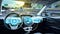source

There is a lot of hype going around on making vehicles autonomous through Artificial Intelligence. Self-driving cars not only serve as an entertainment wow-factor but they also have the ability to prevent anywhere from 75% to 90% of the car accidents we suffer each year. But the question is, how can an AI model be trained to deal with such a complex task of driving?

There are several approaches. And one of these approaches is through Reinforcement Learning. By representing each point in time of the vehicle as a state of the environment (S_t), the directions that the car could maneuver in and other control dynamics as its actions (A_t), and creating a reward model for the vehicle to learn from its mistakes (R_t+1), we can build a simple self-driving car simulator that will learn to drive effectively on its own!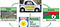A simplified model of simulation a self-driving car through Reinforcement Learning

But hold on…

Obviously, it isn’t that simple when it comes to simulating self-driving cars in the real world. But, by combining other Machine Learning algorithms with further research on reward models and other metrics, Reinforcement Learning will become a key player in manufacturing autonomous vehicles.

One researcher even created an End-to-End Reinforcement Learning Algorithm for simulating a self-driving car through a method called Deep Q-learning. Cool right?

For the purposes of this article, we will focus on understanding the fundamental concepts of the Q-learning model in order to get an intuitive feel for how the ideas of states, actions, and rewards will come into play towards training an agent to play simple video games all the way up to simulating self-driving cars.

Now let’s get technical…

# What is Q-learning?

Q-learning is a model-free reinforcement learning algorithm that attempts to find the most optimal value function [V_π(s)] in order to estimate the best policy (π) so that our agent can maximize the expected return (G_t) in a given environment. If you are unfamiliar with these terms, don’t worry we will learn about them in the following sections.

Sidenote… If you are a bit shaky on the fundamental concepts of Reinforcement Learning such as states, actions, rewards and the different components of an MDP, check out the following articles that will get you up to speed!

To intuitively understand this algorithm, we must break down the four underlying concepts for Q-learning and other Reinforcement Learning algorithms that you will continue to see over and over as you dive deeper into this field — returns, policies, value functions, and the Bellman Equations.

# Returns

The return gives us the sum of all of the future rewards from a given state. It can either be finite given that the scope of the problem is episodic with fixed time steps or infinite if our environment is continuous. The return is acquired through the following equation and is denoted as G_t:The equation for the return

Big T is the final time step that will either end the episode or can either be infinite if the task is continuous. But wait… if big T is infinite, doesn’t that mean G_t will also be infinite?

Absolutely correct.

In order to combat this problem, we multiply each reward by a hyperparameter called the discount factor (γ). The discount factor will be between 0 and 1 and will exponentially grow for future rewards in order to reduce their impact on our current calculations. In essence, the discount factor accounts for uncertainty in the future. Super philosophical, isn’t it?

Therefore, the previous equation will turn into the following:The equation for the discounted return

By multiplying by the discount factor, it allows for G_t to converge to a certain value even if big T approaches infinity.

We can further simplify this process: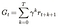The simplified equation for the discounted return

Instead of throwing equations at you and leave you questioning, let’s analyze this concept of returns and discount factors through a simple visual consisting of three states.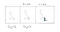A simple deterministic environment with three states

For simplicity's sake, the environment above is deterministic and our agent can only move right starting from state S_0. Let’s calculate the return without the discount factor for both S_0 and S_1: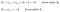Mapping the returns for the states in the environment (no discount factor)

We see that both returns equal 2. Since they are the same, it’s ambiguous as to which state is better for our agent to be in. However, by simply observing the environment, it’s clear that being in state S_1 is better than S_0 since it’s closer to the reward of +2. In order to better represent the returns, let’s calculate it again but including the discount factor. Since it’s a hyperparameter, let’s set the discount factor equal to 0.9.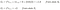Mapping the returns for the states in the environment (with discount factor)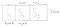value of the return by calculating with the discount factor

By using the discount factor, we can now clearly see that because S_1 has a higher value than S_0, the more optimal state for our agent to be in is state S_1. Therefore, the discount factor allows the return to work in environments that are never-ending + maps out a sequentially appropriate path that our agent can follow to choose the best actions.

But how can the agent choose its actions?

Policies!

# Policies

Recall that policies in reinforcement learning indicate the probability of choosing an action (a) given our current state (s) and is denoted by the following equation:The equation for the policy

Note: A(s) represents all of the actions that our agent can take from a given state (s).

Because policies are given by the probability distribution over all actions from the state (s), we can derive that the sum of all of the policies from a given state should equal one.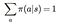the sum of the policies from a current state equals one

However, one notable difference is that policies are completely different from state-transition probabilities. Due to environments usually being stochastic, it’s possible that even if we choose an action (left) to get to some state (s_a), the environment may force our agent to move towards state (s_b) contradicting the policy that we chose. Therefore, the probability of choosing an action =! the probability of transitioning to a successor state given that the environment is either stochastic or non-deterministic. Therefore, it’s important to remember that…

• Policies map states (s) to actions (a).
• State-transition probabilities map current states (s) to next states (s_t+1) .

Now, that we have defined the policy, let’s play around a bit more with the math.The equation for the immediate next reward

R^a_{ss’} outputs the immediate next reward that was acquired given that our agent has already chosen an action (a) and has travelled to the successor state (s’) from the current state (s).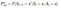The equation for the state-transition probability

P^a_{ss’} is the state-transition probability for travelling to the successor state (s’).

Let’s understand how all of these ideas connect through a visual representation of a simple environment with four states.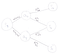A visual representation of the connections between policies and state-transition probabilities

In this world, our agent will be starting at state S_1 and can either take action a_x or action a_y and travel to three states in total. As we can clearly see from this diagram that choosing an action comes before our agent maneuvering to the next state.

Let’s say we wanted our agent to travel to state S_a. We would then program our agent to follow policy π(a_x | s_1) in order to reach the correct successor state. However, there is still a chance that our agent could end up in state S_b given the state transition probabilities that are attached to action a_x. Through this example, it’s clear that the policy is connected to the state-transition probability, but they are not equal to each other.

Overall, our goal to “solve the reinforcement learning problem” is to find the most optimal policy that will give our agent the highest return.

However, in q-learning, our agent will not know the policy in the first place. For this reason, we will have to use a concept called value functions in order to help us find the policy.

# Value Functions

There are two types of value functions— state value functions and action-value functions. In essence, value functions answer the question, “so what’s next from here?”

## State Value Function

The state value function quantifies how beneficial it is for our agent to be in a particular state. Hold on, you might be thinking why do we need to value each state when we already have rewards for each state?

The answer to that question is that unlike immediate rewards (r_{t+1}), value functions hold the sum of all expected future rewards given the current state. Wait… what else includes the sum of future rewards?

The return! Yes, the return is closely tied to the idea of value functions.

However, unlike the return, the value function includes the expected sum of future rewards for all states in the state space in order to account for the stochasticity of environments.

Furthermore, value functions are subscripted by π because they depend on the policy that the agent chooses to follow. Therefore, the equation becomes:The equation for the State-value function

It’s important to keep in mind that value functions following different policies will contain different outputs because the agent’s behaviour (captured by the actions that it chooses to take given by the policy) will vastly influence the calculated values for each state.

# Action-Value Function

Action-value functions quantify how beneficial it is for our agent to take a particular action. This function is also called the q-function that will input state- pairs (s, a) and output the value called the q-value for choosing a particular action.

The q-function is defined as the expected return given the current state and action for all states in the state space and for all actions in the action space.The equation for the Q-function

Furthermore, we can see that a relationship between the state-value function and q-value function exists through the following equation: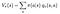Relationship between state-value function and q-function

All this equation says is that the state-value function is a weighted sum of the q-values of the actions that the agent can take from the current state. This notion can be presented in the following diagram: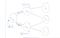Visual representation of the state-value and q-function relationship

Calculating the expected return for an environment with four states would be fairly easy. However, what if there were a thousand states? Calculating the entire return to determine the value function and q-function for each of those thousand states would be far too computationally expensive. Now the question arises, how can we calculate the value function and q-function in an efficient manner?

Simple. We will use The Bellman Equation.

# The Bellman Equation

The Bellman Equation is one of the most fundamental concepts in solving Reinforcement Learning problems. It is an idea taken from dynamic programming that allows us to find the most optimal value functions and policies in a computationally effective manner to solve reinforcement learning problems. There are two Bellman Equations—one for the state-value function and the other for the q-function.The Bellman Equation for the state-value function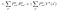The Bellman Equation for the q-function

Ok… not so simple. But wait!

If you were like me, you were probably extremely intimidated after first being introduced to this mathematical madness. However, after understanding how each variable builds on top of each other, understanding these equations will feel much easier and more fun!

To facilitate comprehension, we are going to derive each equation step-by-step in order to get an intuitive feel for how these equations work.

## The State-value function Bellman Equation

Consider the following relationship in the return.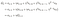The equation for the return to highlight the recursive nature

The return can be broken up into the immediate reward added to the discounted expected return in the next time step. By writing the return in this manner, we can take advantage of its recursive nature when calculating the Bellman Equation for value functions.

Given the previous diagram, Let’s formalize the state-value function Bellman Equation for state s_1 through its original equation: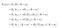Derivation of the State-value function Bellman Equation

As we can see, the original value function breaks up into the expected immediate reward plus the discount value of the next state. We can further expand this equation by multiplying the policies and state transition probabilities in order to capture all variables that will affect the value function for s_1.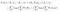The Bellman Equation for the Value Function

From the final expansion, we can see that the original equation does indeed formalize towards the Bellman Equation for the state value function.

Let’s use the following diagram to understand what the sums signify: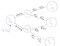Visual representation of the Bellman Equation for the state-value function

The value for state s_1 is equal to the weighted sum of choosing the two actions, receiving the rewards and transitioning into the next state plus the discounted expected value of the next state. Another way to look at this diagram mathematically is that the variables in the lines represent the probabilities (policies for the action, and the state-transition probability of the successor states) that will be multiplied to the actions and the corresponding states.

Through this model, we can spot the recursive nature of the Bellman Equation since the value of the successor state will also need to be calculated. This recursive property of the Bellman Equation is what allows us to train our q-learning model in a computationally effective manner since we don’t need to perform a full look ahead into all of the future rewards.

## The Q-function Bellman Equation

We can follow a similar process for deriving the Bellman Equation for the q-function. Let’s assume that our agent has now chosen action a_x. Therefore, the derivation would be as follows: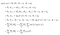Derivation for the Q-function Bellman Equation

Again this equation can be visually represented as the expected immediate reward + the expected discounted value of the successor state.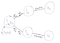Visual representation of the Bellman Equation for the q-function

Take some time, and let these relationships sink into your brain. Once you find that it clicks, the concepts will become fairly intuitive.

Personally, I find that thinking of these calculations as just multiplying a bunch of sticks (the probabilities) by the circles (states and actions) helps me understand how policies, value functions and the corresponding Bellman Equations are interlinked.

However, we still haven’t figured out the optimal policy and value function yet. Next time, we will see how we can turn the Bellman Equation into the Bellman’s Optimality Equation which we will then use to find the optimal policy and train our q-learning model.

# Key Takeaways

• Q-learning is a model-free Reinforcement Learning algorithm
• Returns, policies, value functions, and the Bellman Equations are four of the most fundamental concepts in Q-learning and other RL algorithms
• The return represents the discounted sum of future rewards.
• Policies calculate the probability of taking an action from a given state
• Value functions quantify the goodness of being in a state or taking an action.
• The Bellman Equations can be used to find the most optimal policies and value functions in a computationally effective manner.

## The Startup

Get smarter at building your thing. Join The Startup’s +724K followers.

## The Startup

Get smarter at building your thing. Follow to join The Startup’s +8 million monthly readers & +724K followers.

Written by

## Tawsif Kamal

A 17 y/o Reinforcement Learning enthusiast looking to leverage tech towards driving social change 🤖## The Startup

Get smarter at building your thing. Follow to join The Startup’s +8 million monthly readers & +724K followers.

## More From Medium

Medium is an open platform where 170 million readers come to find insightful and dynamic thinking. Here, expert and undiscovered voices alike dive into the heart of any topic and bring new ideas to the surface. Learn more

Follow the writers, publications, and topics that matter to you, and you’ll see them on your homepage and in your inbox. Explore

If you have a story to tell, knowledge to share, or a perspective to offer — welcome home. It’s easy and free to post your thinking on any topic. Write on Medium